# Why using a Policy-based algorithm instead of Deep Q-learning?

### Why Using a Policy-Based algorithm Instead of Deep Q-learning?

#### A super-simple explanation about Policy Gradient.Photo by Gradient on Unsplash

I assume you are familiar with Q-learning and deep Q-learning concepts. We find Q-values as the expected sum of rewards given a state and action in the last method. So we may use a Tabular method to store all Q(s, a) or train an approximator like a neural network for mapping state and actions to Q-values. To choose which action to take given a state, we take the action with the highest Q-value (the maximum expected future reward I will get at each state).

So Deep Q-learning is so cool! Why do we need another method? Scientists try to find another way to approach RL problems to call policy-based. In this method, they try to find the best policy in an environment instead of finding Q-values and then acting greedy.

Policy-based methods have better convergence properties. They just follow a gradient to find the best parameters so we’re guaranteed to converge on a local maximum (worst case) or global maximum (best case). Besides, policy gradients are more effective than Tabular methods. While policy concludes action, Tabular methods should calculate Q-values for all actions. Imagine you have continuous action or so many options to choose from.

A third advantage is that policy gradients can learn a stochastic policy, while value functions can’t. It means that you choose between actions using a distribution. Choose a1 with 40%, a2 with 20%, and …. So you have wider policy space to search on. Feel free to read about the benefits of stochastic policies over deterministic ones. For example, imagine this little environment. In the gray blocks, you either should go right or left. When you have a deterministic policy, our agent gets stuck. But in a stochastic one, the agent may choose right or left within a distribution. So it will not be stuck and will reach the goal state with high probability.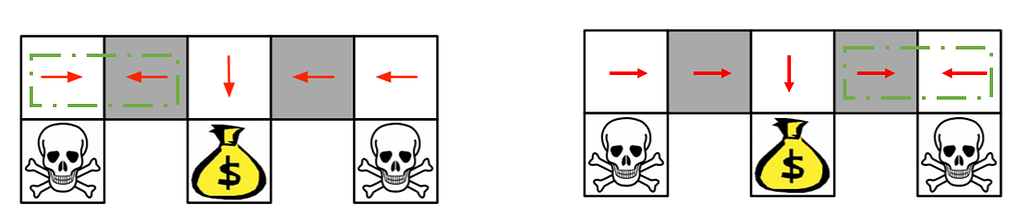agent gets stuck in gray blocks(from David Silver lectures https://www.davidsilver.uk/teaching/ )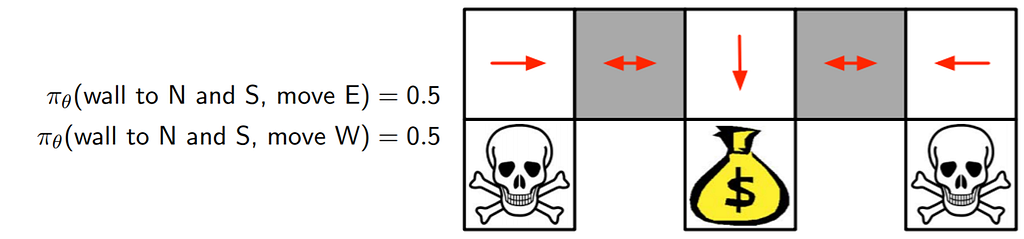using stochastic policy you may not be stuck as you choose actions from a distribution. (from David Silver lectures https://www.davidsilver.uk/teaching/)

Until now, we understand another type of algorithm with some benefits over deep Q-learning called policy gradient, which follows gradient rules to find parameters map state to optimal action.

So how we should search in policy space? Our choice is good if it maximizes the expected sum of rewards.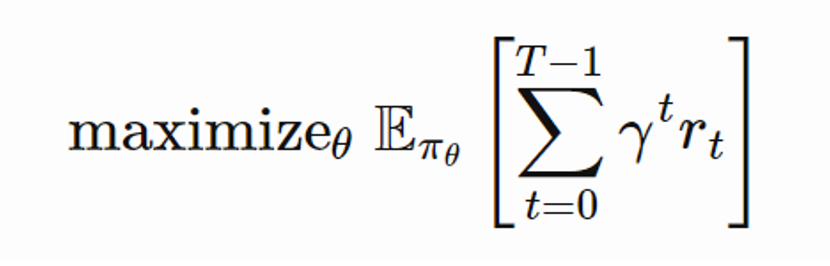So in episodic environments, the discounted sum of rewards means to return from starting point. Imagine you always start from s0 then expected reward from s0 using that policy is your J. You can rewrite the above formula as: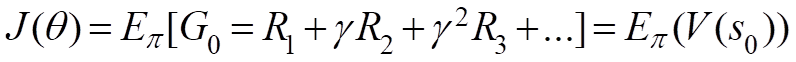If you can’t rely on a specific start state then you may use the average value. You may weight average over V(s) for different where the weights are the probability of starting from that state (or the probability of the occurrence of the respected state.)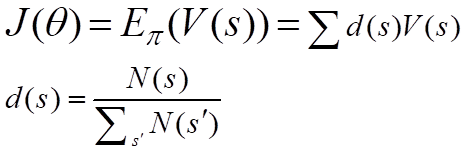Now you can rewrite V(s) as weighted average on expected rewards where weights are the probability of choosing specific action.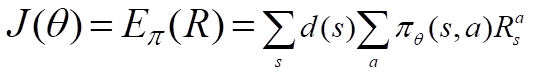Now we have our objective function we should use gradient ascent(opposite of gradient descent) to maximize J.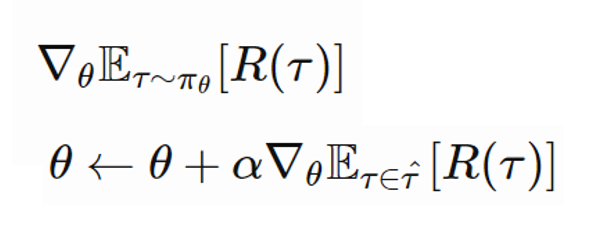Here we need 2 lemmas first.

Lemma 1: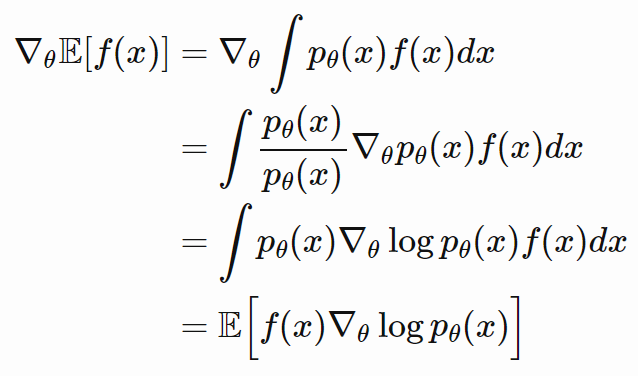Lemma 2: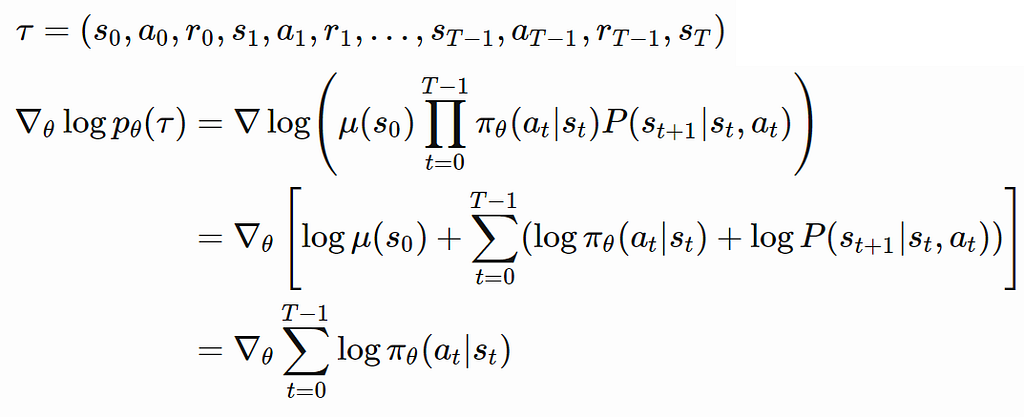Combine these 2 lemmas with our objective function, we can compute the gradient of J. So now gradient only applies our policy which can be modeled using a neural network.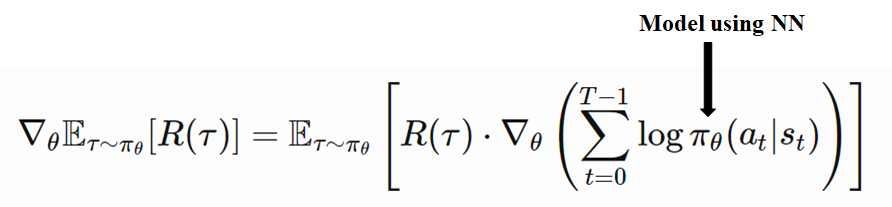Write it as a simple equation our final gradient policy approach is called REINFORCE.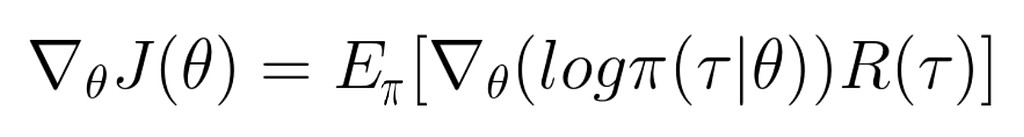do you see any problem here?!

Here is the Policy Gradient method all in on formula! To wrap up I put the algorithm from Sutton's book: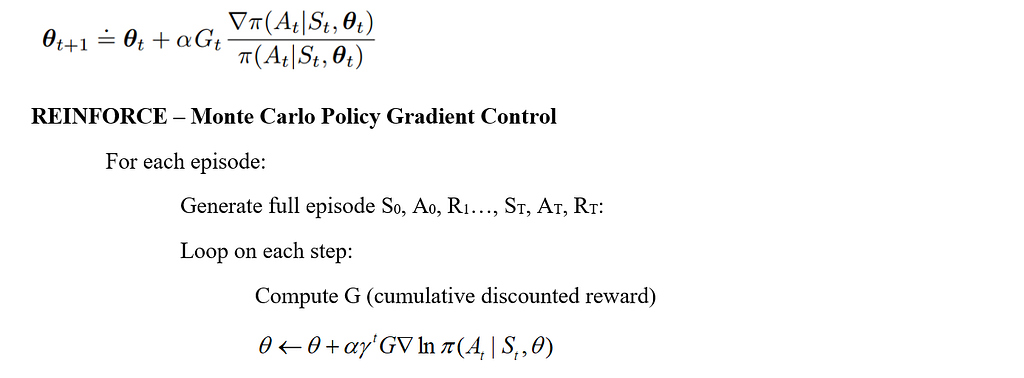REINFORCE method

But there is a little problem. We use R in our objective so we should know the cumulative reward at end of the episode. It is kind of obeys Monte Carlo rules. Wait until the agent finishes the episode and then change parameters and update policy. Why this is important? Well if you make a wrong action middle of the episode but the episode overall obtains success then you think all actions were good enough. It means you can not recognize if an action negatively affects the episode while you see overall effects. So maybe instead of R, you can use the expected reward you may get from that state and action.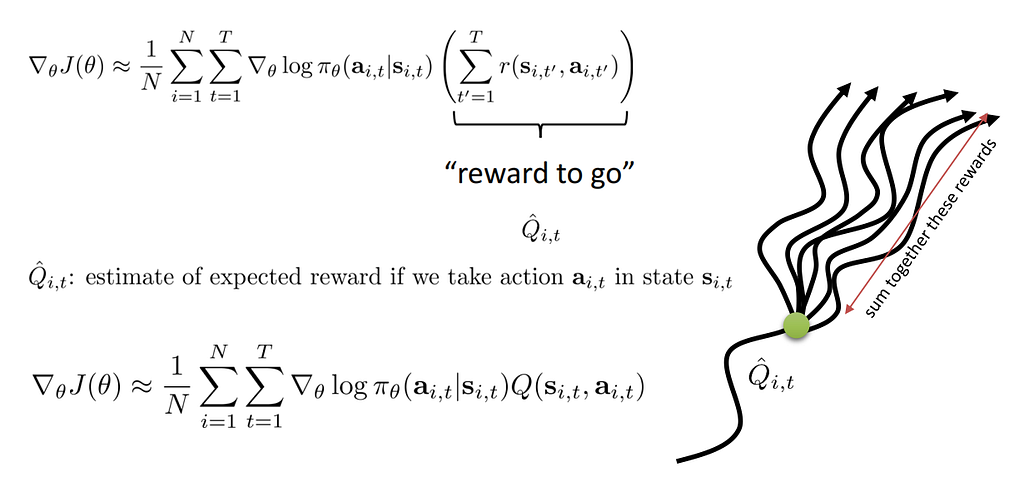After this change, you should now estimate Q-value too. It’s the second approach call Actor critic methods. We will cover this Topic in another story. Be sure you understand the path we go through step by step.Why using a Policy-based algorithm instead of Deep Q-learning? was originally published in Towards AI on Medium, where people are continuing the conversation by highlighting and responding to this story.

Published via Towards AI